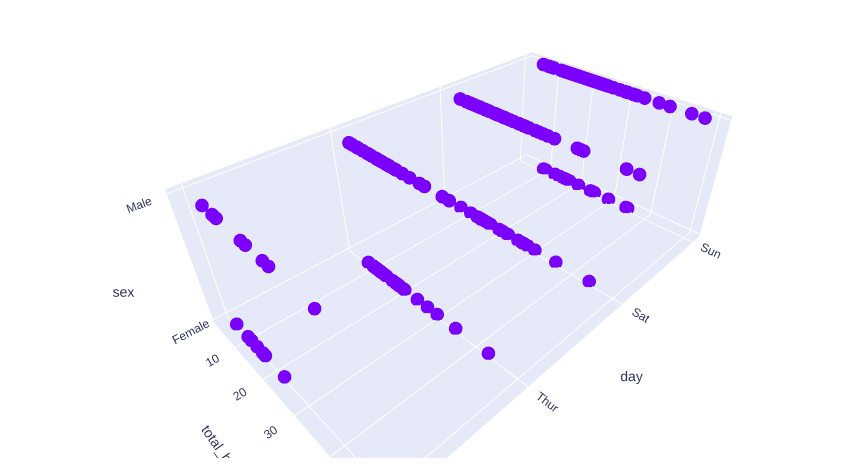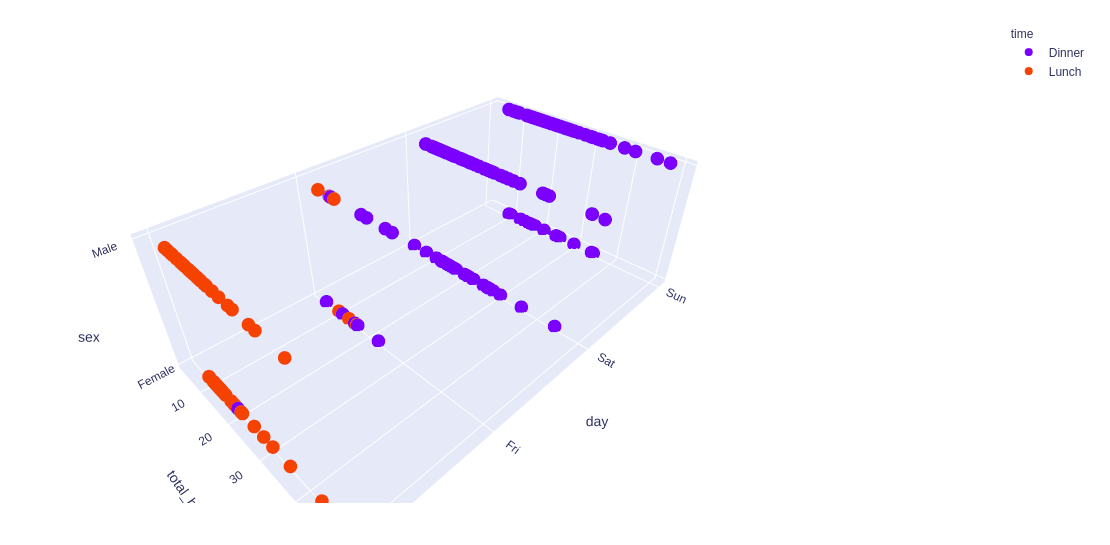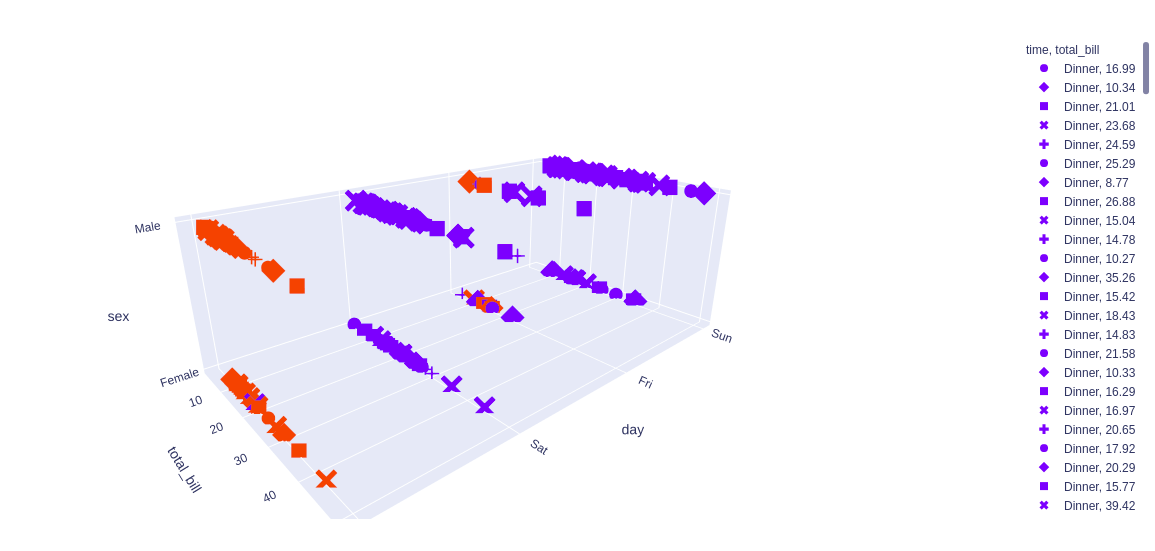Open in App
Not now

# plotly.express.scatter_3d() function in Python

• Last Updated : 17 Jul, 2020

Plotly library of Python can be very useful for data visualization and understanding the data simply and easily. Plotly graph objects are a high-level interface to plotly which are easy to use.

## plotly.express.scatter_3d() function

This function is used to create a 3D scatter plot and can be used with pandas dataframes. Each row of dataframe is represented by a symbol mark in 3D space in a scatter plot.

Syntax: plotly.express.scatter_3d(data_frame=None, x=None, y=None, z=None, color=None, symbol=None, size=None, text=None, hover_name=None, hover_data=None, custom_data=None, error_x=None, error_x_minus=None, error_y=None, error_y_minus=None, error_z=None, error_z_minus=None, animation_frame=None, animation_group=None, category_orders={}, labels={}, size_max=None, color_discrete_sequence=None, color_discrete_map={}, title=None, template=None, width=None, height=None)

Parameters:

data_frame: DataFrame or array-like or dict needs to be passed for column names.

x, y, z: This parameters is either a name of a column in data_frame, or a pandas Series or array_like object. Values from this column or array_like are used to position marks along the x, y and z axis in cartesian coordinates respectively.

color: This parameters assign color to marks.

symbol: This parameter is used to assign symbols to marks. It is either a name of a column in data_frame, or a pandas Series or array_like object.

size: This parameter is used to assign mark sizes. It is either a name of a column in data_frame, or a pandas Series or array_like object.

hover_name:  Values from this column or array_like appear in bold in the hover tooltip.

hover_data: This parameter is used to appear in the hover tooltip or tuples with a bool or formatting string as first element, and list-like data to appear in hover as second element Values from these columns appear as extra data in the hover tooltip.

Example 1:

## Python3

 `# Python program to demonstrate 3D scatter``# plot`` ` `import` `plotly.express as px`` ` `df ``=` `px.data.tips()`` ` `plot ``=` `px.scatter_3d(df, x ``=` `'day'``, ``                     ``y ``=` `'total_bill'``,``                     ``z``=``'sex'``)``plot.show()`

Output:Example 2: Using color argument

## Python3

 `# Python program to demonstrate scatter``# plot`` ` `import` `plotly.express as px`` ` `df ``=` `px.data.tips()`` ` `plot ``=` `px.scatter_3d(df, x ``=` `'day'``, ``                     ``y ``=` `'total_bill'``,``                     ``z ``=` `'sex'``, ``                     ``color ``=` `'time'``)``plot.show()`

Output:Example 3:

## Python3

 `# Python program to demonstrate scatter``# plot`` ` `import` `plotly.express as px`` ` `df ``=` `px.data.tips()`` ` `plot ``=` `px.scatter_3d(df, x ``=` `'day'``, ``                     ``y ``=` `'total_bill'``, ``                     ``z ``=` `'sex'``, ``                     ``color ``=` `'time'``,``                     ``symbol ``=` `'total_bill'``)``plot.show()`

Output:My Personal Notes arrow_drop_up